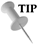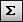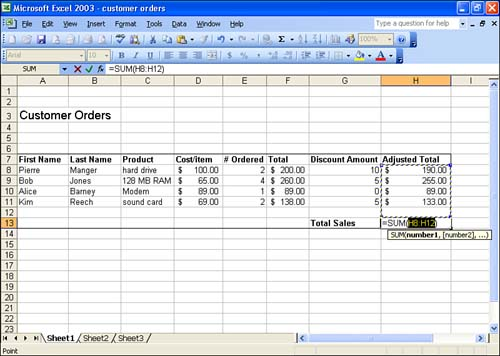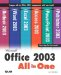# What Are Functions?

You already learned in Lesson 3, "Performing Simple Calculations," how to create your own formulas in Excel. When you work with more complex calculations, you are better off using Excel's built-in formulasfunctions.

Functions are ready-made formulas that perform a series of operations on a specified range of values. For example, to determine the sum of a series of numbers in cells A1 through H1, you can enter the function =SUM(A1:H1). Excel functions can do all kinds of calculations for all kinds of purposes, including financial and statistical calculations.

Every function consists of the following three elements:

• The = sign, which indicates that what follows is a function (formula).

• The function name , such as SUM, that indicates which operation will be performed.

• A list of cell addresses, such as (A1:H1), which are to be acted upon by the function. Some functions can include more than one set of cell addresses, which are separated by commas (such as A1,B1,H1).How Ranges Are Designated When a range of cells (a contiguous group of cells) is referenced in Excel, the format A1:H1 is used. This tells you that the range of cells starts at A1 and includes all the cells from A1 to (:) and including cell H1. We look at cell ranges in more detail in Lesson 11, "Working with Ranges."

You can enter functions into the worksheet by typing the function and cell references (as you did with your own formulas), or you can use the Insert Function feature, which walks you through the process of creating a function in a worksheet (you will work with the Insert Function feature in a moment). Table 5.1 lists some of the Excel functions that you will probably use most often in your worksheets.

##### Table 5.1. Commonly Used Excel Functions

Function

Example

Description

AVERAGE

=AVERAGE(B4:B9)

Calculates the mean or average of a group of cell values.

COUNT

=COUNT(A3:A7)

Counts the number of cells that hold values in the selected range or group of cells. This can also be used to tell you how many cells are in a particular column, which tells you how many rows are in your spreadsheet.

IF

=IF(A3>=1000,"BONUS","NO BONUS")

Allows you to place a conditional function in a cell. In this example, if A3 is greater than or equal to 1000, the true value, BONUS, is used. If A3 is less than 1000, the false value, NO BONUS, is placed in the cell.

MAX

=MAX(B4:B10)

Returns the maximum value in a range of cells.

MIN

=MIN(B4:B10)

Returns the minimum value in a range of cells.

PMT

=PMT(.0825/12,360,180000)

Calculates the monthly payment on a 30-year loan (360 monthly payments) at 8.25% a year (.0825/12 a month) for \$180,000.

SUM

=SUM(A1:A10)

Calculates the total in a range of cells.Specify Text with Quotation Marks When you are entering text into a function, the text must be enclosed within quotation marks. For example, in the function =IF(A5>2000,"BONUS","NO BONUS"), if the condition is met (the cell value is greater than 2000), the word BONUS will be returned by the function. If the condition is not met, the phrase NO BONUS will be returned in the cell by the function.

Excel provides a large number of functions listed by category. There are Financial functions, Date and Time functions, Statistical functions, and Logical functions (such as the IF function described in Table 5.1). The group of functions that you use most often depends on the type of worksheets you typically build. For example, if you do a lot of accounting work, you will find that the Financial functions offer functions for computing monthly payments, figuring out the growth on an investment, and even computing the depreciation on capital equipment.

Although some commonly used functions have been defined in Table 5.1, as you become more adept at using Excel you might want to explore some of the other functions available. Select Help, Microsoft Excel Help . On the Contents tab of the Help window, open the Function Reference topic. Several subtopics related to Excel functions and their uses are provided.

#### Using AutoSum

Adding a group of cells is probably one of the most often-used calculations in an Excel worksheet. Because of this fact, Excel makes it very easy for you to place the SUM function into a cell. Excel provides the AutoSum button on the Standard toolbar. AutoSum looks at a column or row of cell values and tries to select the cells that should be included in the SUM function.

To use AutoSum, follow these steps:

1. Select the cell where you want to place the SUM function. Typically, you will choose a cell that is at the bottom of a column of values or at the end of a row of data. This makes it easy for AutoSum to figure out the range of cells that it should include in the SUM function.

2.Click the AutoSum button on the Standard toolbar. AutoSum inserts =SUM and the cell addresses that it thinks should be included in the function (see Figure 5.1).

##### Figure 5.1. AutoSum inserts the SUM function and selects the cells that will be totaled by the function.3. If the range of cell addresses that AutoSum selected is incorrect, use the mouse to drag and select the appropriate group of cells.

4. Press the Enter key. AutoSum calculates the total for the selected range of cells.Quick AutoSum To bypass the step where Excel displays the SUM formula and its arguments in the cell, select the cell in which you want the sum inserted and double-click the AutoSum button on the Standard toolbar.Microsoft Office 2003 All-in-One
ISBN: B005HKSHB2
EAN: N/A
Year: 2002
Pages: 660
Authors: Joe Habraken

Similar book on Amazon